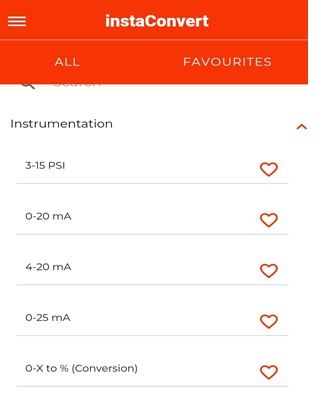# InstaConvert the best calculator app for instrumentation and electrical engineers (FREE)#### InstaConvert – Instrumentation and Electrical tool

Instaconvert is a calculator and converter app that will be very useful for instrumentation and electrical engineers. It can do most of the electrical and instrumentation calculations. This app can do the flow rate calculation by using the input parameters such as pipe diameter, constructed diameter, measured pressure difference, and it can also do a lot more calculations. We can also do electrical calculations such as calculating the power, power factor, frequency, and a lot more with the help of this app. So this app is capable to do the basic calculations and also the high-end calculations related to the instrumentation and electrical field and that’s why this app will be very useful for engineers.

#### Important features of this app and what makes it better than other apps

• This app can be operated offline and it is free.
• This app won’t collect any of your data, like the photos and videos or other data’s in your mobile
• This app is updated frequently so that you can get new engineering calculations
• We can also save the frequently used conversion or calculation in this app
• We can do the unit conversion by using this app
• On the above-left side we can see a search icon by using this we can choose which conversion and calculation we need to do.

#### Instrumentation tools

• 3-15psi – This will help us to determine the air-pressure range of pneumatic instruments in which, 3 represents the low end and 15 represents the high end. So the instrument pressure range will be mostly between the 3&15 PSI.
• 4-20mA – In a field instrument 4-20mA signal is used to transfer signals from the instrument and sensors and this 4-20mA signal can be considered as 0-100% of a process variable, and this can be calculated.
• 0-x% conversion – it is for measuring the 0-20mA signal converter and by giving the start value and low value we can calculate it
• Linear mA to Square root mA – this can be used for the conversion of 4-20mA linear signal to square root signal and vice-versa
• Bubble level – This can be used to calculate the liquid level by giving the pressure value that is needed to force the gas into the liquid
• Temperature from resistance – by using this we can determine the temperature coefficient of resistance for resistance temperature detectors.
• Open tank – this tool can be used to do the open tank measurement and in the open tank low-pressure cell would be open to the air. The measurement can be done by using the pressure in the liquid and by the gravity in the tank liquid
• Orifice plate error – This tool can be used to determine the orifice plate error and the required inputs for this measurement will be the maximum flow through the orifice plate, the differential pressure at that flow rate, differential pressure measurement errors, and current flow.
• Hydrostatic level – this measurement is also for level measurement and it is done with the help of a submersible pressure transmitter. The required inputs values are maximum upper rate value, minimum lower rate value, process liquid density, transmitter height offset, actual liquid level, and impulse line fluid density.
• Liquid purge – this is also used for level measurement

This app also has other level measurement tools like level measurement two seals, level measurement one seal above, level measurement one seal below, interface wet leg, and also level measurement in a closed vessel.

#### Electrical tools

• OHMs LAW (R) – this is the basic electrical calculation by which we can find the resistance R=V/I

OHM’s LAW (V) – this tool is to measure the voltage and it will be V=I/R

OHM’s LAW (I) – this is to measure the current I=V/R

• Air-core inductance – this tool is to calculate the air core inductor coil inductance and the required inputs are diameter, length, and turns of the coil
• Parallel resistance – this tool can be used to calculate the total resistance of resistors in parallel and the required inputs are the value of the resistors in parallel
• LC resonance – this tool is to calculate the resonance of the inductor and capacitor and so by giving the capacitor and inductor value we can calculate the frequency
• Wheatstone – this tool is to calculate the value of the resistor that is missing and we can also do the voltage across the bridge calculation
• Color code resistance – by using this code we can calculate the resistor value by giving the strip color as input.
• Effective tension from HP & FPM– this tool is to calculate the effective tension by using the horsepower and FPM.
• DC motor horsepower – this is to do the horsepower calculation of DC motor and the required inputs for this calculation is voltage, current, and efficiency
• NM to LBIN – this tool is to the conversion of newton meter to pound
• LBIN to NM – this is to do the pound to torque conversion
• DOL & STAR DELTA STARTER – this tool is to calculate the motor torque, fuse, relay, and contractors. The required inputs for these calculations are system voltage, phase, motor size, motor RPM, power factor.

This app has other electrical tools like short circuit calculator, transformer fault calculator, voltage divider, frequency, power factor in three-phase and single-phase, and a lot more.

#### Unit converters

By this, we can do the conversions of area, force, energy, time, mass, length, temperature, current, volume, pressure, speed, power, charge, and a lot more. An example of this will be the area that can be converted to square mile – hector, or to square yard-acre, and in this manner, we can also do the conversions related to the above-mentioned units.